Question

# How to calculate theoretical yield benzil in the hexaphenylbenzene experiment, with 0.753 g of benzoin produced.(molecular...

How to calculate theoretical yield benzil in the hexaphenylbenzene experiment, with 0.753 g of benzoin produced.(molecular weight of benzil is 210.28 and amount used 100ml.)

Then theoretical yield of tetraphenylcyclopentadienon with 0.264 g of benzil product.

(molecular weight of tetraphenylcyclopentadienone is 384.48 and used 100 mg)

We are given, mass of benzoin = 0.753 g , molar mass of benzil = 210.28 g/mol

The reaction to find theoretical yield benzil is benzoin reacted with cupric acetate to create benzil.

So there is 1:1 mole ratio between benzoin and benzil

Step 1 ) Calculate the moles of benzoin

We know,

Moles = mass / molar mass

= 0.753 moles / 212.24 g.mol-1

= 0.00355 moles

Step 2) Calculating the moles of benzil from benzoin

We know there is mole ration between benzoin and benzil is 1:1

So, moles of benzoin = moles of benzil

So, moles of benzil = 0.00355 moles

Step 3 ) Calculate theoretical yield

Theoretical yield of benzil = 0.00355 moles * 210.28 g/mol

= 0.746 g

When benzil and 1,3 diphenylacetone reacted with CH3OH, [C6H5CH2N(CH3)3]+, OH- to create tetraphenylcyclopentadienone.

So, moles of benzil = moles of tetraphenylcyclopentadienon

So, moles of tetraphenylcyclopentadienon = 0.00355 moles

So theoretical yield of tetraphenylcyclopentadienon = 0.00355 moles * 384.48 g/mol

= 1.36 g

#### Earn Coins

Coins can be redeemed for fabulous gifts.

Similar Homework Help Questions
• ### 7. Calculate the maximum amount of hexaphenylbenzene (molar mass = 534.7 g/mol) possible (theoretical yield) when 1...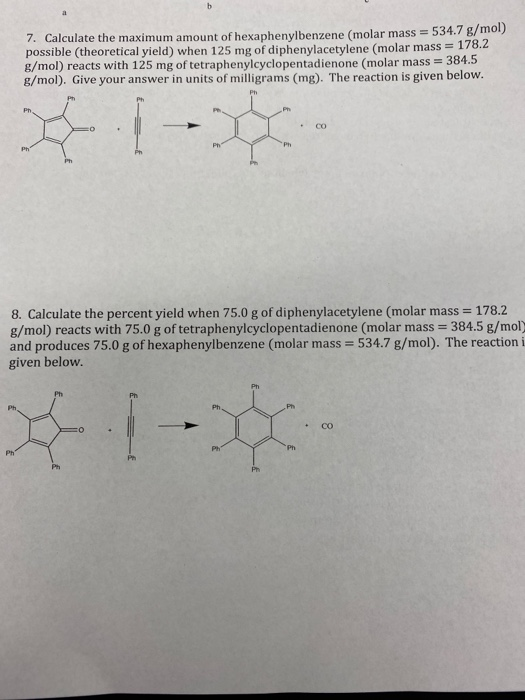7. Calculate the maximum amount of hexaphenylbenzene (molar mass = 534.7 g/mol) possible (theoretical yield) when 125 mg of diphenylacetylene (molar mass - 178.2 g/mol) reacts with 125 mg of tetraphenylcyclopentadienone (molar mass 384.5 g/mol). Give your answer in units of milligrams (mg). The reaction is given below. 8. Calculate the percent yield when 75.0 g of diphenylacetylene (molar mass = 178.2 g/mol) reacts with 75.0 g of tetraphenylcyclopentadienone (molar mass = 384.5 g/mol) and produces 75.0 g of hexaphenylbenzene...

• ### 5. Select the incorrect statement. (2 pts) a) An NMR of of benzoin will have a...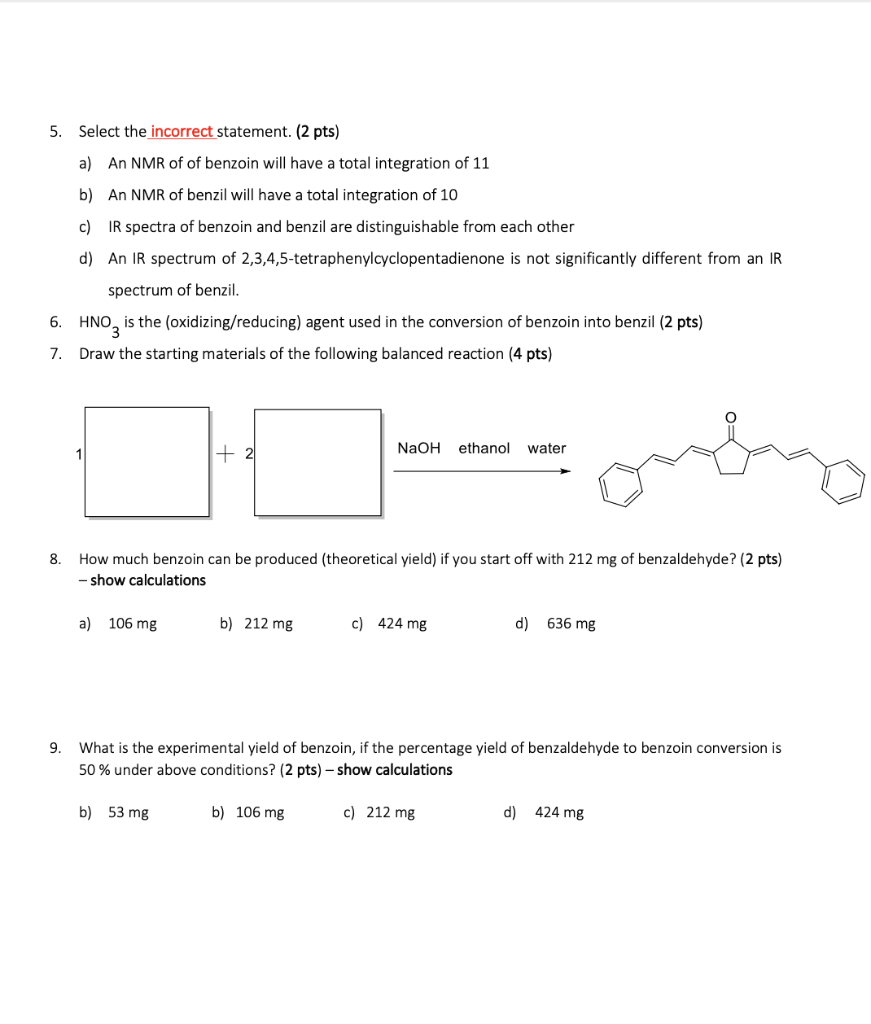5. Select the incorrect statement. (2 pts) a) An NMR of of benzoin will have a total integration of 11 b) An NMR of benzil will have a total integration of 10 c) IR spectra of benzoin and benzil are distinguishable from each other d) An IR spectrum of 2,3,4,5-tetraphenylcyclopentadienone is not significantly different from an IR spectrum of benzil. 6. HNO is the (oxidizing/reducing) agent used in the conversion of benzoin into benzil (2 pts) 7. Draw the starting...

• ### calculate the theoretical yield and percentage yield of 0.053 g of benzil reacted with 0.09 g...

calculate the theoretical yield and percentage yield of 0.053 g of benzil reacted with 0.09 g of NaBH4 (excess) in order to form 1R, 2S- meso hydrobenzoin the actual amount of product obtained is 0.406 g

• ### benzoin: .40g and 1.5 ml of nitric acid, to prepare benzil. you isolated .260g of pure...

benzoin: .40g and 1.5 ml of nitric acid, to prepare benzil. you isolated .260g of pure benzil product after recrystallization. (Density of HN03= 1.42 g/mL) 1. write a balanced chemical equation for the reaction 2. create a stoichiometry table and calculate the limiting reagent in this reaction 3. calculate the theoretical yield and percent yield of the product

• ### Assume your starting with 10.324 grams of benzil for 1 1. Calculate the theoretical yield of your predicted product (2,...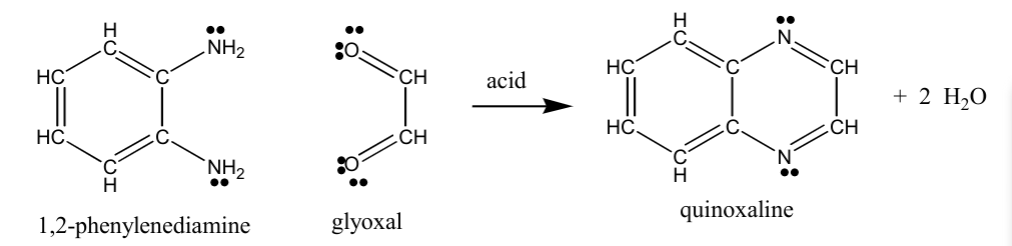Assume your starting with 10.324 grams of benzil for 1 1. Calculate the theoretical yield of your predicted product (2,3-diphenylquinoxaline) 2. Write the balanced overall equation of benzaldehyde to 2,3-diphenylquinoxaline. Combine the three equations of Benzaldehyde --> Benzoin, Benzoin --> Benzil, and Benzil --> 2,3-diphenylquinoxaline using structural representations IÓ _NH2 Ñ c HC CH "CH acid + 2 H2O HC ZCZ CH HC CH 2C 'N NH2 UI N quinoxaline 1,2-phenylenediamine glyoxal

• ### Post lab Exp 10: Preparation of benzil from benzoin Place 1 g of benzoin and 3...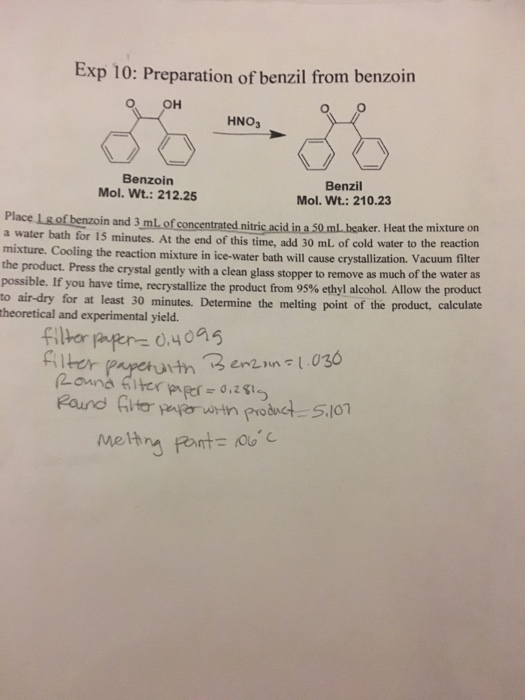Post lab Exp 10: Preparation of benzil from benzoin Place 1 g of benzoin and 3 mL of concentrated nitric acid in a 50 mL beaker. Heat the mixture on a water bath for 15 minutes. At the end of this time, add 30 mL of cold water to the reaction mixture. Cooling the reaction mixture in ice-water bath will cause crystallization. Vacuum filter the product. Press the crystal gently with a clean glass stopper to remove as much of...

• ### >>> QUESTION: Calculate the mass and volume of 150 mmol of benzaldehyde, and the theoretical yields of benzoin,...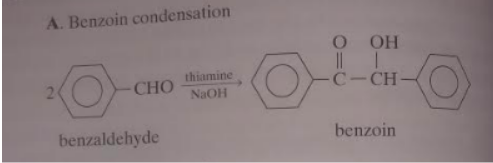>>> QUESTION: Calculate the mass and volume of 150 mmol of benzaldehyde, and the theoretical yields of benzoin, benzyl, and benzilic acid expected from that much benzaldehyde. You will find mol molecular weight below! Reactions and molar ratios: Phyiscal properties: A. Benzoin condensation OOH 20 CHO thiamine NaOH Tc-culo - --CH- -CHO benzaldehyde benzoin B. Oxidation of benzoin OOH C-CH + NH NO, benzil C. Benzilic acid rearrangement Он о potassium benzilate ОН 0 -с-с-он + кс | | benzilic...

• ### In the synthesis of benzil from bonzoin, how do we calculation % yield? For example, when...

In the synthesis of benzil from bonzoin, how do we calculation % yield? For example, when 4 g of benzil was produced from 10 g of benzoin, what is the % yield? Show all your work

• ### How to calculate theoretical yield & actual yield for this problem. A student used 3 mL...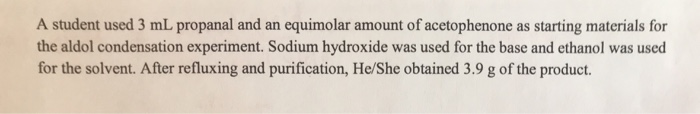How to calculate theoretical yield & actual yield for this problem. A student used 3 mL propanal and an equimolar amount of acetophenone as starting materials for the aldol condensation experiment. Sodium hydroxide was used for the base and ethanol was used for the solvent. After refluxing and purification, He/She obtained 3.9 g of the product.

• ### According to his prelaboratory theoretical yield calculations, a student’s experiment should have produced 1.33 g of...

According to his prelaboratory theoretical yield calculations, a student’s experiment should have produced 1.33 g of magnesium oxide. When he weighed his product after reaction, only 1.05 g of magnesium oxide was present. What is the student’s percent yield?